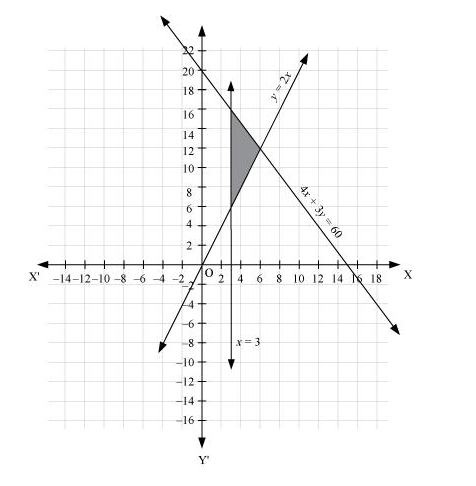# Solve the following system of inequalities graphically:

Question:

Solve the following system of inequalities graphically:

4x + 3y ≤ 60, y ≥ 2xx ≥ 3, xy ≥ 0

Solution:

$4 x+3 y \leq 60 \ldots(1)$

$y \geq 2 x \ldots$ (2)

$x \geq 3 \ldots$ (3)

The graph of the lines, 4x + 3y = 60, y = 2x, and x = 3, are drawn in the figure below.

Inequality (1) represents the region below the line, 4x + 3y = 60 (including the line 4x + 3y = 60). Inequality (2) represents the region above the line, y = 2(including the line y = 2x). Inequality (3) represents the region on the right hand side of the line, x = 3 (including the line x = 3).

Hence, the solution of the given system of linear inequalities is represented by the common shaded region including the points on the respective lines as follows.